### Home > MC2 > Chapter 7 > Lesson 7.2.2 > Problem7-79

7-79.

Sketch each angle below on your paper, and label each one with its measure in degrees.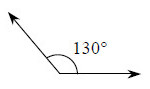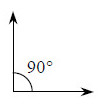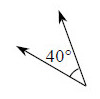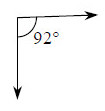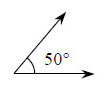1. Identify whether each angle is an acute, obtuse, or right angle.

Recall that acute angles are angles that measure less than $90°$, obtuse angles measure more than $90°$, and right angles measure exactly $90°$.

2. Which angles could form a complementary pair?

Complementary angles are two angles that add up to $90°$.
For example, a $30°$ angle and a $60°$ angle are complementary because $30°+ 60° = 90°$.

3. Which angles could form a supplementary pair?

Supplementary angles are angles that add up to $180°$.
For example, $110°$ and $70°$ are supplementary angles because $110° + 70° = 180°$.